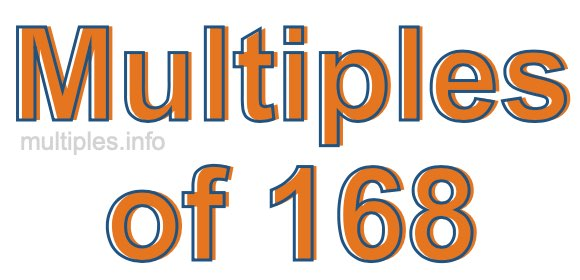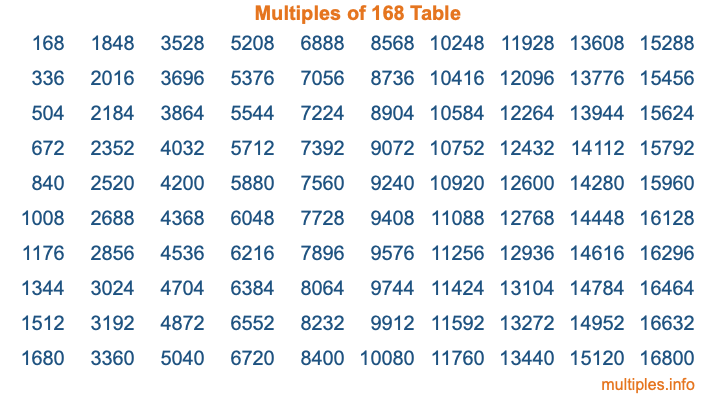Multiples of 168Welcome to the Multiples of 168 page. Here we will first teach you everything you will ever need to know about the multiples of 168, and then give you a study guide summary of everything we taught you to make sure you remember it all. Use this page to look up facts and learn information about the multiples of 168. This page will make you a multiples of one hundred sixty-eight expert!

Definition of Multiples of 168
Multiples of 168 are all the numbers that when divided by 168 equal an integer. Each of the multiples of 168 are called a multiple. A multiple of 168 is created by multiplying 168 by an integer.

Therefore, to create a list of multiples of 168, you start with 1 multiplied by 168, then 2 multiplied by 168, then 3 multiplied by 168, and so on for as long as you want. Thus, the list of the first five multiples of 168 is 168, 336, 504, 672, and 840. To see a larger list of multiples of 168, see the printable image of Multiples of 168 further down on this page. We also have a category where you can choose any nth multiple of 168.

Multiples of 168 Checker
The Multiples of 168 Checker below checks to see if any number of your choice is a multiple of 168. In other words, it checks to see if there is any number (integer) that when multiplied by 168 will equal your number. To do that, we divide your number by 168. If the the quotient is an integer, then your number is a multiple of 168.

Is  a multiple of 168?

Least Common Multiple of 168 and ...
A Least Common Multiple (LCM) is the lowest multiple that two or more numbers have in common. This is also called the smallest common multiple or lowest common multiple and is useful to know when you are adding our subtracting fractions. Enter one or more numbers below (168 is already entered) to find the LCM.

Check out our LCM Calculator if you need more details about the Least Common Multiple or if you need the LCM for different numbers for adding and subtraction fractions.

nth Multiple of 168
As we stated above, 168 is the first multiple of 168, 336 is the second multiple of 168, 504 is the third multiple of 168, and so on. Enter a number below to find the nth multiple of 168.

th multiple of 168

Multiples of 168 vs Factors of 168
168 is a multiple of 168 and a factor of 168, but that is where the similarities end. All postive multiples of 168 are 168 or greater than 168. All positive factors of 168 are 168 or less than 168.

Below is the beginning list of multiples of 168 and the factors of 168 so you can compare:

Multiples of 168: 168, 336, 504, 672, 840, etc.

Factors of 168: 1, 2, 3, 4, 6, 7, 8, 12, 14, 21, 24, 28, 42, 56, 84, 168

As you can see, the multiples of 168 are all the numbers that you can divide by 168 to get a whole number. The factors of 168, on the other hand, are all the whole numbers that you can multiply by another whole number to get 168.

It's also interesting to note that if a number (x) is a factor of 168, then 168 will also be a multiple of that number (x).

Multiples of 168 vs Divisors of 168
The divisors of 168 are all the integers that 168 can be divided by evenly. Below is a list of the divisors of 168.

Divisors of 168: 1, 2, 3, 4, 6, 7, 8, 12, 14, 21, 24, 28, 42, 56, 84, 168

The interesting thing to note here is that if you take any multiple of 168 and divide it by a divisor of 168, you will see that the quotient is an integer.

Multiples of 168 Table
Below is an image of the first 100 multiples of 168 in a table. The table is in chronological order, column by column. The first column has the first ten multiples of 168, the second column has the next ten multiples of 168, and so on.The Multiples of 168 Table is also referred to as the 168 Times Table or Times Table of 168. You are welcome to print out our table for your studies.

Negative Multiples of 168
Although not often discussed or needed in math, it is worth mentioning that you can make a list of negative multiples of 168 by multiplying 168 by -1, then by -2, then by -3, and so on, to get the following list of negative multiples of 168:

-168, -336, -504, -672, -840, etc.

Multiples of 168 Summary
Below is a summary of important Multiples of 168 facts that we have discussed on this page. To retain the knowledge on this page, we recommend that you read through the summary and explain to yourself or a study partner why they hold true.

There are an infinite number of multiples of 168.

A multiple of 168 divided by 168 will equal a whole number.

168 divided by a factor of 168 equals a divisor of 168.

The nth multiple of 168 is n times 168.

The largest factor of 168 is equal to the first positive multiple of 168.

168 is a multiple of every factor of 168.

168 is a multiple of 168.

A multiple of 168 divided by a divisor of 168 equals an integer.

168 divided by a divisor of 168 equals a factor of 168.

Any integer times 168 will equal a multiple of 168.

Multiples of a Number
Here you can get the multiples of another number, all with the same attention to detail as we did for multiples of 168 on this page.

Multiples of
Multiples of 169
Did you find our page about multiples of one hundred sixty-eight educational? Do you want more knowledge? Check out the multiples of the next number on our list!